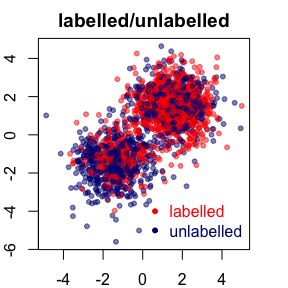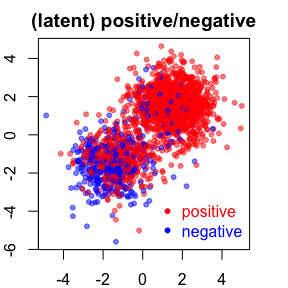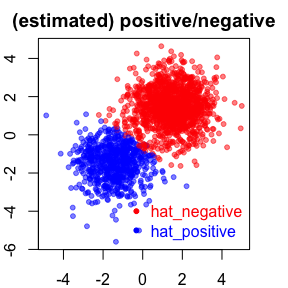# Introduction

PUlasso is an algorithm for parameter estimation and classification using Positive and Unlabelled(PU) data. More concretely, presented with two sets of sample such that the first set consisting of $$n_l$$ positive and labelled observations and a second set containing $$n_u$$ observations randomly drawn from the population with only covariates not the responses being observed and the true positive prevalence information $$P(Y=1)$$, PUlasso algorithm models the relationship between the probability of a response being positive and $$(x, \theta)$$ using the standard logistic regression model:$$P_\theta(y=1|x) := 1/(1+exp(-\theta^Tx))$$ and solves the following optimization problem: $\begin{equation}\label{objFunc} \underset{\theta}{\operatorname{argmin}} -\log L(\theta;x,z) + P_\lambda(\theta) \end{equation}$ where $$\log L(\theta;x,z)$$ is an observed log-likelihood based on covariates and labels $$(x,z)$$, and $$P_\lambda(\theta)$$ is $$\ell_1$$ or $$\ell_1/\ell_2$$ penalty. For more detailed discussion, please see our paper https://arxiv.org/abs/1711.08129

# Basic usage of PUlasso package

We demonstrate basic usage of PUlasso package using simulated PU data.

data("simulPU")

This loads simulPU object which contains the input matrix $$X$$, labels $$z$$, true (latent) responses $$y$$, and the positive prevalence true$$PY1$$. We first visualize the data. We plot the first two columns $$X1-X2$$ colored by $$z$$ or $$y$$ as the first two variables are set to be active in the simulation. From the following plots, we see that many positive samples are marked as unlabelled. For more details about the simulation setting, do help(simulPU)We fit the model using the most basic call. By default it fits the model for 100 values of $$\lambda$$ starting from the null model with lasso penalty.

(fit=grpPUlasso(X=simulPU$X,z=simulPU$z,py1=simulPU$truePY1)) ## ## Call: grpPUlasso(X = simulPU$X, z = simulPU$z, py1 = simulPU$truePY1)
##
## Optimization Method:  "CD"
##
## Max nIters:  1000

coefficients can be extracted using coef function. Here we extract estimated coefficients for 30th $$\lambda$$. By default, coefficients are returned in an original scale. If desired, we can set std.scale=T to obtain coefficients in the standardizeds scale.

coef(fit, lambda=fit$lambda) ## [,1] ## (Intercept) 0.05544055 ## X1 0.30819669 ## X2 0.36584501 ## X3 0.00000000 ## X4 0.00000000 ## X5 0.00000000 If we want to predict responses at certain $$x$$, we use the predict function. By default, it returns estimated probabilities. xnew = matrix(rnorm(10),2,5) predict(fit,newdata = xnew,lambda = fit$lambda)
##           [,1]
## [1,] 0.5914671
## [2,] 0.6460069

It is a common practice to choose $$\lambda$$ based on cross-validation. Main function for the k-fold cross-validation is cv.grpPUlasso.

(cv.fit = cv.grpPUlasso(X=simulPU$X,z=simulPU$z,py1=simulPU$truePY1)) ## ## Call: cv.grpPUlasso(X = simulPU$X, z = simulPU$z, py1 = simulPU$truePY1)
##
## Optimization Method:  "CD"
##
## Max nIters:  1000

We use deviance for a measure of model fit. Average deviance and standard error of deviance over all $$k$$-folds for all $$\lambda$$ values saved in cv.fit$cvm and cv.fit$cvsd, respectively. We are particularly interested in two lambda values : lambda.min which gives the minimum mean cross-validation deviance, and lambda.1se which corresponds to the largest $$\lambda$$ such that cvm is within one standard error of the minimum. We can also extract coefficients corresponding to such lambda levels.

coef(cv.fit,lambda=cv.fit$lambda.1se) ## [,1] ## (Intercept) 0.31212314 ## X1 0.41972292 ## X2 0.51936892 ## X3 0.00000000 ## X4 0.10378309 ## X5 -0.02988138 We finalize this section by demonstrating how to do a classification based on fitted models. A natural threshold of .5 is applied for a classification. We plot $$X1-X2$$ colored by $$\hat{y}$$ to check classification performances. phat<-predict(cv.fit,newdata = simulPU$X,lambda = cv.fit$lambda.1se,type = "response") yhat<-1*(phat>0.5)## Group Penalty We can also use a group lasso penalty. Suppose $$X1$$ is in group 1, $$X2-X3$$ are in group 2, and $$X4-X5$$ are in group 3. We only need to provide a membership information using an additional vector, here named as a grpvec. grpvec = c(1,2,2,3,3) fit.grp = grpPUlasso(X=simulPU$X,z=simulPU$z,py1=simulPU$truePY1,group = grpvec)

All members in the group are either all included or not included. For example, we see from 13th $$\lambda$$ to 14th $$\lambda$$, members in group 2 are entered the model together.

coef(fit.grp,fit.grp$lambda[12:15]) ## [,1] [,2] [,3] [,4] ## (Intercept) -0.02699613 -0.02687234 -0.031632001 -0.036079220 ## X1 0.32136211 0.33761227 0.341612439 0.342443679 ## X2 0.00000000 0.00000000 0.018538324 0.039900657 ## X3 0.00000000 0.00000000 -0.001038037 -0.002210257 ## X4 0.00000000 0.00000000 0.000000000 0.000000000 ## X5 0.00000000 0.00000000 0.000000000 0.000000000 ## Fitting with a sparse matrix PUlasso can exploit a sparsity in an input matrix for a more efficient calculation. If dgCMatrix type of the input matrix is provided, PUlasso automatically performs a sparse calculation. For a simple demonstration, here we create dgCMatrix objects based on $$X$$. First we create a sparse matrix sparseX by imposing 0 on 95% of the entries of $$X$$. sparseX <- simulPU$X
sparseX[sample(1:length(simulPU$X),size = length(simulPU$X)*0.95)]<-0
sparseX<-Matrix(sparseX)
class(sparseX)
##  "dgCMatrix"
## attr(,"package")
##  "Matrix"

Those input matrices can be used in the same way. For example,

(spfit<-grpPUlasso(sparseX,simulPU$z,simulPU$truePY1))
##
## Call:  grpPUlasso(X = sparseX, z = simulPU$z, py1 = simulPU$truePY1)
##
## Optimization Method:  "CD"
##
## Max nIters:  1000
newx = matrix(rnorm(10),2,5)
predict(spfit,newdata = newx,lambda = spfit$lambda) ## [,1] ## [1,] 0.4806071 ## [2,] 0.4662717 ## Optimization By default, PUlasso uses a block-coordinate descent algorithm to solve optimization problem . However, it provides other optimization options such as proximal full gradient descent(GD), stochastic gradient descent(SGD), and variants of SGD including stochastic variance-reduced gradient(SVRG) and stochastic average gradient(SAG). For example, using SVRG method, we fit, (fit.SVRG = grpPUlasso(X=simulPU$X,z=simulPU$z,py1=simulPU$truePY1,method="SVRG",eps = 1e-6,lambda =fit$lambda)) ## ## Call: grpPUlasso(X = simulPU$X, z = simulPU$z, py1 = simulPU$truePY1,      lambda = fit\$lambda, eps = 1e-06, method = "SVRG")
##
## Optimization Method:  "SVRG"
##
## Max nIters:  20000

By default, PUlasso does 10*number of observations iterations. The algorithm terminates early if the difference of current parameter value from the previous one is less than the eps.